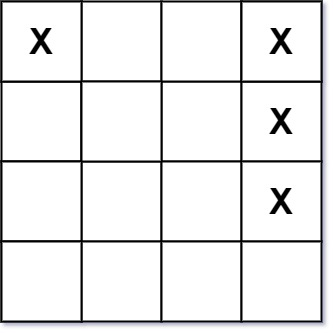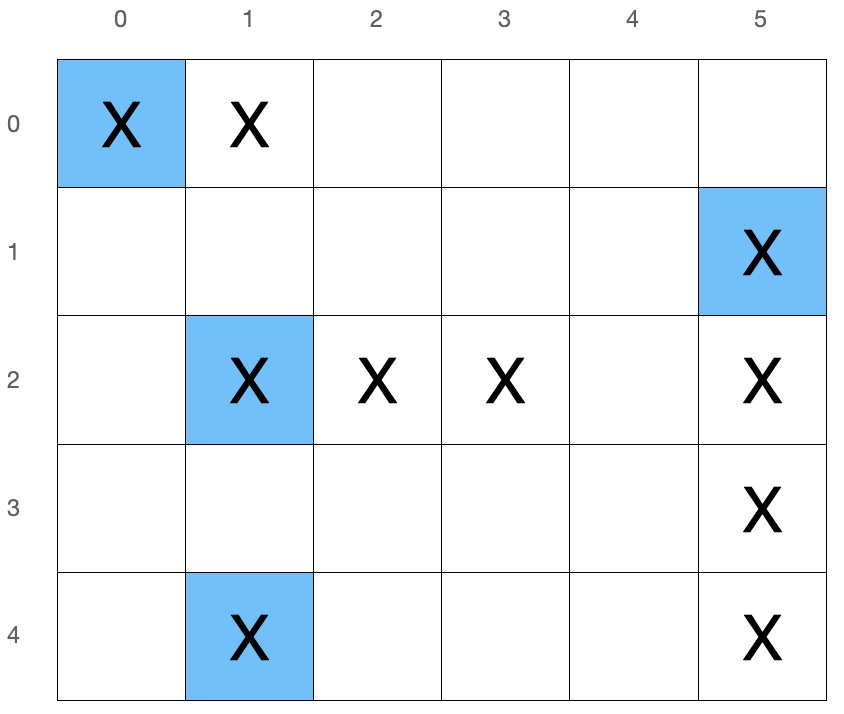# LeetCode 419. Battleships in a Board Solution

Given an m x n matrix board where each cell is a battleship 'X' or empty '.', return the number of the battleships on board.

Battleships can only be placed horizontally or vertically on board. In other words, they can only be made of the shape 1 x k (1 row, k columns) or k x 1 (k rows, 1 column), where k can be of any size. At least one horizontal or vertical cell separates between two battleships (i.e., there are no adjacent battleships).

Example 1:Input: board = [["X",".",".","X"],[".",".",".","X"],[".",".",".","X"]] Output: $2$

Example 2:

Input: board = [["."]]
Output: $0$

Constraints:

• m == board.length
• n == board[i].length
• $1 \leq m, n \leq 200$
• board[i][j] is either '.' or 'X'.

## Full Solution

To count the number of battleships, we can first observe that each battleship acts the same as an island described in this problem. We can run the solution to this same problem however it's unnecessary and a much more elegant solution exists.

The problem statement mentions that each battleship will be a 1 x k or k x 1 rectangle and no battleships are adjacent. Instead of counting battleships, we can pick a cell in each battleship that defines it. Let's call this the leader of the ship. To solve the problem, we can simply count the number of leaders. The total number of leaders we count will be the same as the number of battleships.

For each battleship, we will let the leader be the leftmost and upmost cell out of all cells in that battleship.

### ExampleIn this specific example, there are four battleships with leaders (indicated with blue) located at (0,0), (2,1), (4,1), and (1,5).

How can we determine efficiently if a cell is a leader?

Since the leader is located in the top-left cell of a battleship and no two battleships are adjacent, we can observe that a cell with an 'X' is a leader if the cells to the left (board[i][j-1]) and above (board[i-1][j]) it (if they exist) are not 'X' cells. In addition, cells that are not leaders will always have an 'X' cell to the left or above it so they will never be counted. To count the total number of leaders, we'll iterate through all cells and count the number of cells that satisfy the condition mentioned above.

### Time Complexity

We can check if a cell is a leader in $\mathcal{O}(1)$ and since there are $\mathcal{O}(MN)$ cells, our time complexity is $\mathcal{O}(MN)$.

Time Complexity: $\mathcal{O}(MN)$

### Space Complexity

Since we only maintain a counter for the number of leaders, our space complexity is $\mathcal{O}(1)$.

Space Complexity: $\mathcal{O}(1)$

## C++ Solution

1class Solution {
2   public:
3    int countBattleships(vector<vector<char>>& board) {
4        int ans = 0;
5        int m = board.size();
6        int n = board.size();  // dimensions for board
7        for (int i = 0; i < m; i++) {
8            for (int j = 0; j < n; j++) {
9                if (board[i][j] == 'X') {
10                    if ((i == 0 || board[i - 1][j] == '.') && (j == 0 || board[i][j - 1] == '.')) {
11                        // check if cell is a leader
12                        ans++;
13                    }
14                }
15            }
16        }
17        return ans;
18    }
19};

## Java Solution

1class Solution {
2    public int countBattleships(char[][] board) {
3        int ans = 0;
4        int m = board.length;
5        int n = board.length; // dimensions for board
6        for (int i = 0; i < m; i++) {
7            for (int j = 0; j < n; j++) {
8                if (board[i][j] == 'X') {
9                    if ((i == 0 || board[i - 1][j] == '.') && (j == 0 || board[i][j - 1] == '.')) {
10                        // check if cell is a leader
11                        ans++;
12                    }
13                }
14            }
15        }
16        return ans;
17    }
18}

## Python Solution

1class Solution:
2    def countBattleships(self, board: List[List[str]]) -> int:
3        ans = 0
4        m = len(board)
5        n = len(board)  # dimensions for board
6        for i in range(m):
7            for j in range(n):
8                if board[i][j] == "X":
9                    if (i == 0 or board[i - 1][j] == ".") and (
10                    	j == 0 or board[i][j - 1] == "."):
11                        # check if cell is a leader
12                        ans += 1
13        return ans
14